JEE  >  JEE Main Practice Test- 26

# JEE Main Practice Test- 26 - JEE

Test Description

## 90 Questions MCQ Test JEE Main & Advanced Mock Test Series - JEE Main Practice Test- 26

JEE Main Practice Test- 26 for JEE 2023 is part of JEE Main & Advanced Mock Test Series preparation. The JEE Main Practice Test- 26 questions and answers have been prepared according to the JEE exam syllabus.The JEE Main Practice Test- 26 MCQs are made for JEE 2023 Exam. Find important definitions, questions, notes, meanings, examples, exercises, MCQs and online tests for JEE Main Practice Test- 26 below.
Solutions of JEE Main Practice Test- 26 questions in English are available as part of our JEE Main & Advanced Mock Test Series for JEE & JEE Main Practice Test- 26 solutions in Hindi for JEE Main & Advanced Mock Test Series course. Download more important topics, notes, lectures and mock test series for JEE Exam by signing up for free. Attempt JEE Main Practice Test- 26 | 90 questions in 180 minutes | Mock test for JEE preparation | Free important questions MCQ to study JEE Main & Advanced Mock Test Series for JEE Exam | Download free PDF with solutions
 1 Crore+ students have signed up on EduRev. Have you?
JEE Main Practice Test- 26 - Question 1

### If a, b, c are in H.P., then straight line x/a + y/b + 1/c = 0 always passes thro' a fixed point and that point is

Detailed Solution for JEE Main Practice Test- 26 - Question 1

The correct option is C.
Since.a, band c are in HP. Then 1/a, 1/b and 1/c are in AP. 2/b = 1/a + 1/c ⇒ 1/a - 2/b + 1/c = 0 Hence, straight line x/a + y/b + 1/c = 0 always passes through a fixed point (1, -2).

JEE Main Practice Test- 26 - Question 2

### The angle between the line (x - 2)/2 = (y + 1)/-1 = (z - 3)/2 and the plane 3x + 6y - 2z + 5 = 0 is

Detailed Solution for JEE Main Practice Test- 26 - Question 2

D is the correct option.sin⁻1(4/21)

JEE Main Practice Test- 26 - Question 3

### If a,b,c are any three vectors, the statement is true

Detailed Solution for JEE Main Practice Test- 26 - Question 3

D is the correct option.a.(b-c)=a.b-a.c is correct if a,b,c are any three vectors.

JEE Main Practice Test- 26 - Question 4

The equilibrium constant in a reversible chemical reaction at a given temperature

Detailed Solution for JEE Main Practice Test- 26 - Question 4

The correct option is C.
The equilibrium constant in a reversible reaction at a given temperature. Solution : Equilibrium constant is independent of original concentration of reactant.

JEE Main Practice Test- 26 - Question 5

The structure of white phosphorus is

Detailed Solution for JEE Main Practice Test- 26 - Question 5

C is the correct option.The structure of white phosphorus is tetrahedral.
White phosphorus, yellow phosphorus or simply tetraphosphorus (P4) exists as molecules made up of four atoms in a tetrahedral structure. The tetrahedral arrangement results in ring strain and instability. The molecule is described as consisting of six single P–P bonds.

JEE Main Practice Test- 26 - Question 6

Isopropyl chloride undergoes hydrolysis by

Detailed Solution for JEE Main Practice Test- 26 - Question 6

The correct is C.
Isopropyl chloride undergoes hydrolysis via both SN-1 and SN-2 mechanisms. Both of them yield the same result/ products. Since, isopropyl chloride is a secondary alkyl halide.

JEE Main Practice Test- 26 - Question 7

An organic compound A reacts with methyl magnesium iodide to form an addition product which on hydrolysis forms the compound B. Compound B gives blue colour salt in Victor Meyer's test. The compounds A and B are respectively.

Detailed Solution for JEE Main Practice Test- 26 - Question 7

The correct option is C.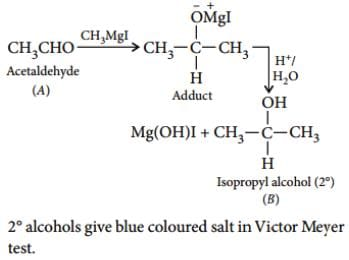JEE Main Practice Test- 26 - Question 8

The area formed by triangular shaped region bounded by the curves y = sinx, y = cos x and x = 0 is

Detailed Solution for JEE Main Practice Test- 26 - Question 8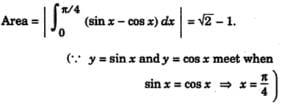JEE Main Practice Test- 26 - Question 9
The integral part of (√2 + 1)6 is
JEE Main Practice Test- 26 - Question 10

The coordinates of the pole of the line lx+my+n=0 with respect to the circle x2+y2=1 are

JEE Main Practice Test- 26 - Question 11
For solving dy/dx=4x+y+1 suitable substitution is
JEE Main Practice Test- 26 - Question 12

The complex numbers z₁,z₂,z₃ satisfying (z₁-z₃)/(z₂-z₃) = 1-(i√3/2) then triangle is

Detailed Solution for JEE Main Practice Test- 26 - Question 12

(z1-z2)/(z2-z3)= cos(-60)+sin(-60)=e-i60

taking mode on both sides

Absolute value of(z1-z2/z2-z3)=1

Therefore |z1-z2|=|z2-z3|

Also angle between z1-z2 & z2-z3 is 60

So given triangle is equilateral triangle.

JEE Main Practice Test- 26 - Question 13

i2 + i4 + i6 + ... + upto (2n + 1) =

JEE Main Practice Test- 26 - Question 14

Integrating factor of dy/dx+y/x = x3-3 is

JEE Main Practice Test- 26 - Question 15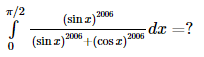Detailed Solution for JEE Main Practice Test- 26 - Question 15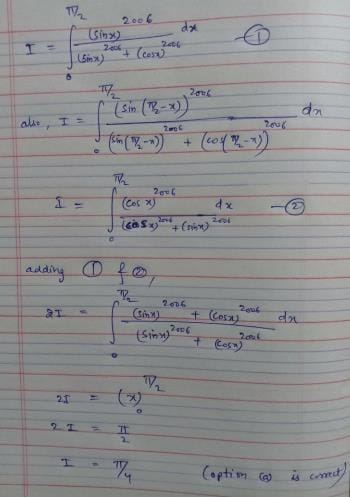JEE Main Practice Test- 26 - Question 16

If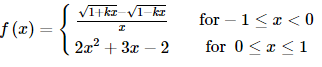is continuous at x = 0, then k =

JEE Main Practice Test- 26 - Question 17

The foci of an ellipse are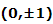and minor axis is of unit length. Then the equation of the ellipse is

Detailed Solution for JEE Main Practice Test- 26 - Question 17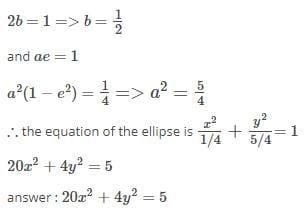JEE Main Practice Test- 26 - Question 18

If y=cot⁻1[(1+x)/(1-x)], (dy/dx)=

Detailed Solution for JEE Main Practice Test- 26 - Question 18

Given y = cot-1 (1+x)/(1-x)

Put x = tan θ

θ = tan-1 x

Then y = cot-1 (1 + tan θ)/(1- tan θ)

y = cot-1 (tan π/4 + tan θ)/(1- tan π/4 tan θ)

= cot-1 tan (π/4 +θ)

= cot-1 cot (π/2 – (π/4 +θ))

= π/4 – θ

= π/4 – tan-1 x

dy/dx = -1/(1+x2)

JEE Main Practice Test- 26 - Question 19

Which statement is logically equivalent to "If Yoda cannot use a lightsaber, then he cannot help Luke win the battle."

Detailed Solution for JEE Main Practice Test- 26 - Question 19

A statement is logically equivalent to its contrapositive.

To form the contrapositive, switch the "If" and  "then" sections

of the statement AND insert "NOTs" into each section.

Notice in this situation, that inserting

"NOTs" turns the thoughts positive (you are negating negative thoughts).

JEE Main Practice Test- 26 - Question 20

In the following question, a Statement of Assertion (A) is given followed by a corresponding Reason (R) just below it. Read the Statements carefully and mark the correct answer-
Assertion(A): If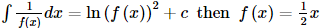Reason(R) : When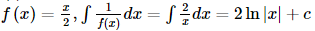JEE Main Practice Test- 26 - Question 21

If f : ℝ → ℝ is defined by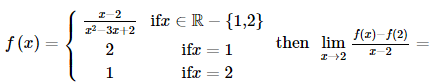JEE Main Practice Test- 26 - Question 22

In the following questions, a Statement of Assertion (A) is given followed by a corresponding Reason (R) just below it. Read the statements carefully and mark the correct answer -
Assertion (A): The sum of the series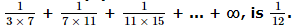Reason (R): The numbers A1, A2, ....., An are said to be the n arithmetic means between two given numbers a and b if a, A1, A2, ...., An, b are in A.P.

JEE Main Practice Test- 26 - Question 23

In the following question, a Statement of Assertion (A) is given followed by a corresponding Reason (R) just below it. Read the Statements carefully and mark the correct answer-
Assertion(A):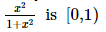Reason (R): If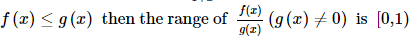Detailed Solution for JEE Main Practice Test- 26 - Question 23

The correct option is Option C.

When f(x) = g(x)

Then the range includes 1.

But it is already given that 1 is excluded.

Hence, the assertion is correct but the reason behind it is false.

JEE Main Practice Test- 26 - Question 24

If A and B are two matrices such that A + B and AB are both defined, then

Detailed Solution for JEE Main Practice Test- 26 - Question 24

Since A+B is defined, A and B are matrices of the same type,
say m×n; Also, AB is defined.
So, the number of columns in A must be equal to the number of rowsin B ie. n=m.
Hence, A and B are square matrices of the same order.

JEE Main Practice Test- 26 - Question 25

In the following question, a Statement-1 is given followed by a corresponding Statement-2 just below it. Read the statements carefully and mark the correct answer-
Let the vectors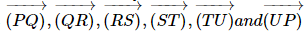represent the sides of a regular hexagon.
Statement-1: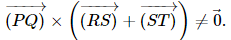.
Statement-2: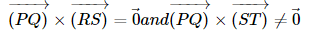JEE Main Practice Test- 26 - Question 26

Let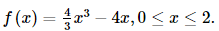Then the global minimum value of the function is

Detailed Solution for JEE Main Practice Test- 26 - Question 26

f ′ (x)   = 4So, for 0 ≤ x < 1, we get f ′ x < 0, i . e . , f x is m.d. and for 1 < x ≤ 2 we get f ′ (x)   > 0, i . e . , f (x)    is m.i .

∴ min f (x)   =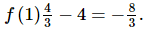JEE Main Practice Test- 26 - Question 27
The mean of distribution is 22.2 and its mode is 23.3. The median is
JEE Main Practice Test- 26 - Question 28

The parabola y2=4x passes thro' the point (2,-6), then the length of its latus rectum is

Detailed Solution for JEE Main Practice Test- 26 - Question 28

It is given that the parabola passes through the point (2,-6)
Length of its latus rectum is 4a
y= 4ax
i.e.

(-6)= 4a(2)
⇒a = 9/2
∴ Length of the latus rectum is 4 x 9/2 = 18

JEE Main Practice Test- 26 - Question 29

If nPr=840, nCr=35, then n=

Detailed Solution for JEE Main Practice Test- 26 - Question 29

We know that ,
1) nPr. = n!/( n - r )!
2) nCr = n!/ [ ( n - r )! r ! ]
3) r! = ( nPr )/ nC

It is given that ,

nPr = 840 ---( 1 )

nCr = 35 ---( 2 )

r! = ( nPr )/ ( nCr )

= 840/35

= 24

r! = 4 × 3 × 2 × 1

r ! = 4!
Therefore,

r = 4

Substitute r value in equation ( 1 ),

We get

n!/ ( n - 4 ) ! = 840

n( n - 1 )( n - 2 )( n - 3 ) = 840

n( n - 1 )( n - 2 )( n - 3 ) = 7× 6 × 5 × 4

Therefore ,

n = 7

JEE Main Practice Test- 26 - Question 30

In a Binomial distribution, the probability of getting a success is 1/4 and standard deviation is 3. Then its mean is

Detailed Solution for JEE Main Practice Test- 26 - Question 30

Let n be successive independent trials and p the probability of getting a success.

The the mean and variance of a binomial distribution is given by :

Mean = np

Variance = np(1 - p)

In this case we have :

p = 1/4

Variance = (standard deviation) ²

Variance = 3² = 9

From this we can get the value of n as follows :

Variance = np(1 - p)

Doing the substitution we have

9 = 1/4n( 1 - 1/4)

9 = 1/4n(3/4)

9 = 3/16n

n = 16/3 × 9

n = 48

Mean = np

Mean = 48 × 1/4 = 12

The mean of the binomial distribution is equal to 12.

JEE Main Practice Test- 26 - Question 31

If 4 sin⁻1x + cos⁻1x = π, then x is equal to

JEE Main Practice Test- 26 - Question 32

The probability distribution of a random variable X is given below. Then its mean is
X = xi 1 2 3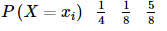JEE Main Practice Test- 26 - Question 33

If A-B=π/4, then (1+tan A)(1-tanB) is equal to

Detailed Solution for JEE Main Practice Test- 26 - Question 33

A-B=π/4

Which implies A=B+π/4

Taking tan on both sides

tanA=tan(B+π/4)

tanA=(tanB + tan(π\4))/(1- tanB.tan(π/4))

tanA=(tanB+1)/(1-tanB)

Substituting the value of tanA in

(1+tanA).(1-tanB) we get

(1+(tanB+1)/(1-tanB)).(1-tanB)

= (1-tanB+tanB+1)/(1-tanB).(1-tanB)

=2

JEE Main Practice Test- 26 - Question 34
If |a|=3, |b|=4, |c|=5 and a+b+c=0, the angle between a and b is
JEE Main Practice Test- 26 - Question 35

The stablest among the following is

JEE Main Practice Test- 26 - Question 36

Addition of a solution of oxalate to an aqueous solution of mixture of Ba2⁺, Sr2⁺ and Ca2⁺ will precipitate

JEE Main Practice Test- 26 - Question 37

What is the lowest energy of the spectral line emitted by the hydrogen atom in the Lyman series ? (h = Planck constant; c = Velocity of light; R = Rydberg constant).

JEE Main Practice Test- 26 - Question 38

The nodal plane in the π-bond of ethene is located in

Detailed Solution for JEE Main Practice Test- 26 - Question 38

Formation of a π - bond occurs by the sideways overlap of p-orbitals of two C-atoms.The molecular plane does not have any π - electron density since the p-orbitals are perpendicular to the plane containing the ethene molecule.The nodal plane in the π-bond of ethene is located in the molecular plane.

JEE Main Practice Test- 26 - Question 39

The compound with carbon uses only its sp3 hybrid orbitals for bond formation is

Detailed Solution for JEE Main Practice Test- 26 - Question 39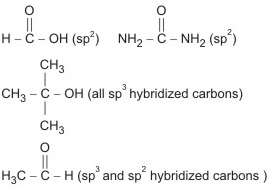JEE Main Practice Test- 26 - Question 40

The hydrolysis of fats and oils in the body produces

Detailed Solution for JEE Main Practice Test- 26 - Question 40

Fats and oils
1. Fats and oils are a concentrated source of energy.
2. Fats and oils are essential for the transport and storage of fat-soluble vitamins in the body.
3. Fats and oils are tri- esters formed from the condensation of glycerol (propane1,2,3-triol) and three carboxylic acid molecules.
4. The hydrolysis products of a fat or oil can be identified from the structure of the fat or oil.

JEE Main Practice Test- 26 - Question 41

Identify the least stable among the following?

Detailed Solution for JEE Main Practice Test- 26 - Question 41

Outer electronic configuration of Li^-1 (2s2 most stable)
Be  ( 2s2 2p1 less stable) than Be (2s2 most stable)
(2s2 2pstable) ; C  (2s2 2p3 most  stable)
As Be  is formed from highly stable configuration it tries to loose electron and form Be.

JEE Main Practice Test- 26 - Question 42

The common features among the species CN⁻, CO and NO⁺ are

Detailed Solution for JEE Main Practice Test- 26 - Question 42

According to Molecular Orbital theory,

CN,CO and NO+ are isoelectronic (14e) and bond order is 3.

JEE Main Practice Test- 26 - Question 43

Heat exchanged in a chemical reaction, at constant temperature and pressure, is called

Detailed Solution for JEE Main Practice Test- 26 - Question 43

Define all the terms to find the correct answer:
(a) Entropy : It is the measurement of randomness or disorder of system.
(b) Enthalpy : It is the total heat content of system or it is the heat which is exchanged in a chemical reaction at constant temperature andpressure.
(c) Free energy : It is the energy of system which can be converted into useful work.
(d) Internal energy : It is the energy stored within a substance.

JEE Main Practice Test- 26 - Question 44

For a reaction 2N₂O₅ → 4NO₂ + O₂ rate and rate constant are 1.02 x 10-4 mol L⁻1 s⁻1 and 3.4 x  10-5 sec⁻1. The conc. of N₂O₅ at that time will be

JEE Main Practice Test- 26 - Question 45

A Carnot engine, working between 500 K to 300 K has a work output of 800 J-cycle⁻1. What is the amount of heat energy supplied to the engine from the source per cycle?

JEE Main Practice Test- 26 - Question 46

An ideal gas heat engine operates in Carnot cycle between 227ºC and 127ºC. It absorbs 6 x 104 cals of heat at higher temp. Amount of heat converted into work is

Detailed Solution for JEE Main Practice Test- 26 - Question 46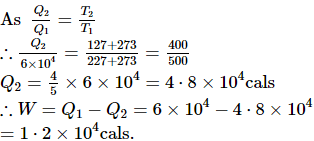JEE Main Practice Test- 26 - Question 47

The densities of graphite and diamond at 298 K are 2.25 and 3.31 g-cm-3 respectively. If the standard free energy difference (ΔGº)is equal to 1895 J-mol-1, then pressure at which graphite will be transformed into diamond at 298 K is

JEE Main Practice Test- 26 - Question 48

Increasing order of stability among the three main conformations(i.e.,Eclipse,Anti,Gauche) of 2-fluorethanol is

Detailed Solution for JEE Main Practice Test- 26 - Question 48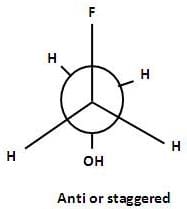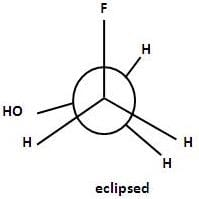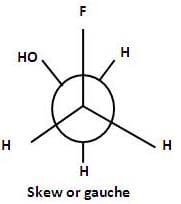The anticonformation is most stable in which F and OH groups are far apart as possible and minimum repulsion between two groups occurs.

In fully eclipsed conformation F and OH groups are so close that the steric strain is maximum .

Hence this conformation is most unstable.

The order of stability of these conformations is anti > gauche > partially eclipsed > fully eclipsed.

Hence a is the correct answer.

JEE Main Practice Test- 26 - Question 49

Eka-aluminium and eka-silicon are known as

Detailed Solution for JEE Main Practice Test- 26 - Question 49

Gallium and Germanium were unknown at the time when Mendeleev's periodic table was formulated. But Mendeleev's predicted the properties of these elements and named them eka-aluminium and eka-silicon. Later when these elements were discovered, their properties were found to be similar to those of aluminium and silicon.

JEE Main Practice Test- 26 - Question 50

The first ionisation potential of Li will be

Detailed Solution for JEE Main Practice Test- 26 - Question 50

As we move from left to right in a period, the ionisation potential increases with increasing atomic number and the ionisation potential keeps on decreasing regularly as we move down a group. Thus the first ionisation potential of Li is less than Be and higher than Na.

JEE Main Practice Test- 26 - Question 51

The CFSE (Crystal Field Stabilisation Energy) for a high spin octahedral complex having d1 configuration is __

Detailed Solution for JEE Main Practice Test- 26 - Question 51

CFSE = [− 0.4p + 0.6q]Δ0
= [− 4p + 6q]Dq
p = electrons in eg orbitals
q = electrons in t2g orbitals
For d1 (high spin) configuration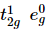CFSE = [- 0.4 x + 0.6 x 0]Δ0
=[−0.40 + 0]Δ0 = − 0.4 Δ
= 4Dq.
(Configuration of d1 in high spin or low spin is same).

JEE Main Practice Test- 26 - Question 52

An aqueous solution of CoCl₂ on addition of excess of concentrated HCl, turns blue due to the formation of

Detailed Solution for JEE Main Practice Test- 26 - Question 52

CoCl2 is a weak Lewis acid, reacting with chloride ion to produce salt containing the tetrahedral [CoCl4]2- ion. CoCl2 is blue when anhydrous, and a deep magneta colour when hydrated, for this reason it is widely used as an indicator for water.

JEE Main Practice Test- 26 - Question 53

The oxidation no. of nitrogen in HNO₃ is

Detailed Solution for JEE Main Practice Test- 26 - Question 53

Oxidation state of N in HNO3 is +5---

OS of H= +1, & O = -2

H = +1
O x 3 = -2 x 3
= -6
net charge on molecule = 0

So +1 -6 +N = 0
=> N = 0+6-1
= +5

JEE Main Practice Test- 26 - Question 54

At cathode ,the electrolysis of aqueous Na₂SO₄ gives

JEE Main Practice Test- 26 - Question 55

Density ratio of O₂ and H₂ molecules is 16:1.The ratio of their r.m.s. velocities will be

Detailed Solution for JEE Main Practice Test- 26 - Question 55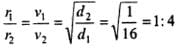JEE Main Practice Test- 26 - Question 56

The property of hydrogen which distinguishes it from other alkali metals is

Detailed Solution for JEE Main Practice Test- 26 - Question 56

Hydrogen is a non-metal while all other members of group 1 (alkali metals) are metals.

JEE Main Practice Test- 26 - Question 57

Low reactivity of vinyl chloride and chlorobenzene is due to :

JEE Main Practice Test- 26 - Question 58

A pyridine derivative (I) reacts with (II), (II) can be a free radical, cation or anion. The structure of the intermediate (III) formed in the reaction is given below, (I) and (II) can be respectively: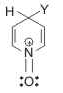JEE Main Practice Test- 26 - Question 59

Bromine water reacts with phenol to form

Detailed Solution for JEE Main Practice Test- 26 - Question 59

if bromine water is added to a solution of phenol in water.

the bromine water is decolourised and a white precipitate is formed which smells of antiseptic .

The precipitate is 2,4,6- tribromophenol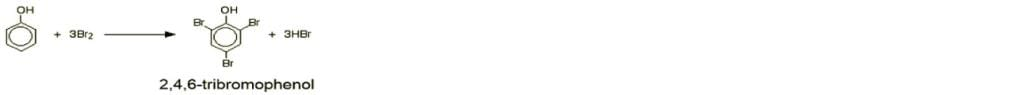Notice the multiple substitution around the ring - into all the activated positions.

JEE Main Practice Test- 26 - Question 60

Conformations are different arrangements of atoms that can be converted into one another by rotation about ___________

Detailed Solution for JEE Main Practice Test- 26 - Question 60

Conformational isomerism is a form of stereoisomerism in which the isomers can be interconverted exclusively by rotations about formally single bonds. Such isomers are generally referred to as conformational isomers or conformers and, specifically, as rotamers.

JEE Main Practice Test- 26 - Question 61

When two deuterium nuclei fuse together in addition to tritium, we get a

JEE Main Practice Test- 26 - Question 62

If a H₂ nucleus is completely converted into energy, the energy produced will be around

Detailed Solution for JEE Main Practice Test- 26 - Question 62

Mass of H2 nucleus =mass of proton =1 amu energy equivalent to 1 amu is 931 MeV so correct option is (b).

JEE Main Practice Test- 26 - Question 63

A uniform resistance wire of length L and diameter d has a resistance R. Another wire of same material has length 4 L and diameter 2 d, the resistance will be

Detailed Solution for JEE Main Practice Test- 26 - Question 63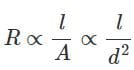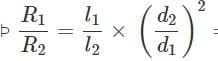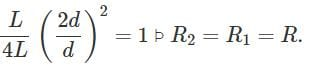JEE Main Practice Test- 26 - Question 64

Nichrome or Manganin is widely used in wire bound standard resistors because of their

JEE Main Practice Test- 26 - Question 65

If particles are moving with same velocity, then maximum de-Broglie's wavelength will be for

Detailed Solution for JEE Main Practice Test- 26 - Question 65

de Broglie wavelength for a particle is given by λ = h/p = h/mv, where m, v and p are the mass, velocity and momentum respectively. it is Planck's constant. Now, since all the particles are moving with same velocity, the particle with least mass will have maximum de-Broglie wavelength. Out of the given four particles (proton, α -particles, i.e. He nucleus and β-particles. i.e. electrons) β-particles has the lowest mass and therefore it has maximum wavelength.

JEE Main Practice Test- 26 - Question 66

When ultraviolet rays are incident on metal plate, then photoelectric effect does not occurs. It occurs by the incidence of

Detailed Solution for JEE Main Practice Test- 26 - Question 66

For photoemission to take place from metal the wavelength of incident ray will be less than threshold value. For photoelectric emission from given metal plate, the incident wavelength must be less than that of ultraviolet rays assuming the wavelength of ultraviolet rays as the threshold value. Out of the given radiations, X-rays have wavelength less than that of ultraviolet rays. Thus, X-rays can cause photoelectric emission.

JEE Main Practice Test- 26 - Question 67

Which rays are not the portion of electro magnetic spectrum?

JEE Main Practice Test- 26 - Question 68

Two point charges +q and -q are held fixed at (-d,0) and (d,0) respectively of a x-y coordinate system. Then

Detailed Solution for JEE Main Practice Test- 26 - Question 68

When you draw the electric field lines for these 2 charges they will starts from the positive charge and end at the negative charge. And when they pass through the Y axis they will be perpendicular to the Y axis, since both the charges are at equal distance and equal in magnitude. The direction of electric field is found by the tangents to the electric field at that point, since the line is parallel to x axis it's slope will also be zero, So the direction of the electric field line will be along the x axis.

JEE Main Practice Test- 26 - Question 69

If 1000 droplets each of charge q and radius r are mixed to form a big drop, then the potential of big drop, as compared to small droplet will be

Detailed Solution for JEE Main Practice Test- 26 - Question 69

If the value of potential on each small drop be V and potential on each small drop be V '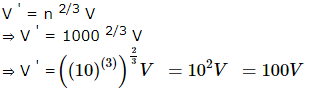JEE Main Practice Test- 26 - Question 70

A satellite with kinetic energy E is revolving around the earth in a circular orbit. The minimum additional kinetic energy required to escape it in the outer space is

Detailed Solution for JEE Main Practice Test- 26 - Question 70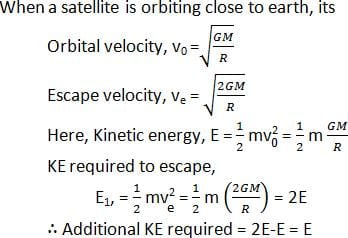JEE Main Practice Test- 26 - Question 71

As we go from the equator to the poles, the value of g

Detailed Solution for JEE Main Practice Test- 26 - Question 71

Since the Earth is flattened at the poles and bulges at the equator, an oblate spheroid the distance from Earth's center to sea level is roughly 21 kilometers (13 miles) greater at the equator than at the poles. Since the value of g varies inversely with the square of the earth's radius, the shorter radius at the pole will result in a greater value of g.

The second reason is that at latitudes nearer the Equator, the outward centrifugal force produced by Earth's rotation is larger than at polar latitudes. This counteracts the Earth's gravity to a small degree – up to a maximum of 0.3% at the Equator – and reduces the value of g at the equator.

So as we move from equator to pole value of g will increase. Hence option C is the correct.

JEE Main Practice Test- 26 - Question 72

On a cold morning, a metal surface will feel colder to touch than a wooden surface because

Detailed Solution for JEE Main Practice Test- 26 - Question 72

Due to higher thermal conductivity of metal than wood, heat begins to flow readily from our body to the metal surface and so we feel colder.

JEE Main Practice Test- 26 - Question 73

A black body at a temperature of 1640 K has the wavelength corresponding to maximum emission equal to 1.75μ. Assuming the moon to be a perfectly black body, the temperature of the moon, if the wavelength corresponding to maximum emission is 14.35 μ is

Detailed Solution for JEE Main Practice Test- 26 - Question 73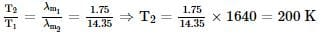JEE Main Practice Test- 26 - Question 74

A man weighs 80 kg. He stands on a weighing scale in a lift, which is moving upwards with a uniform acceleration of 5 m−s⁻2. What would be the reading on the scale?(Take g = 10 m−s⁻2)

Detailed Solution for JEE Main Practice Test- 26 - Question 74

The reading on scale would be
w= M (g+a)
w=80 ( 10+ 5)
w=1200

JEE Main Practice Test- 26 - Question 75

If a car covers 2/5th of the total distance with ν₁ speed and 3/5th distance with ν₂ then average speed is

JEE Main Practice Test- 26 - Question 76

A current passing through a circular coil of two produces a magnetic field of 8 T at its centre. The coil is then rewound, so as to have four turns and current is passed through it is doubled. Now magnetic field at the centre of the coil will be

JEE Main Practice Test- 26 - Question 77

A simple pendulum oscillates in a vertical plane. When it passes through the mean position, the tension in the string is 3 times the weight of the pendulum bob. what is the maximum angular displacement of the pendulum of the string with respect to the vertical ?

Detailed Solution for JEE Main Practice Test- 26 - Question 77

Tension at mean position,

mg+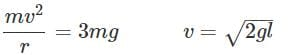(i) and if the body displaces by angle q with the vertical then v =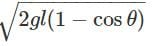(ii) Comparing (i) and (ii),

cosθ = 0 Þ θ = 90º

JEE Main Practice Test- 26 - Question 78

In the following question, a Statement of Assertion (A) is given followed by a corresponding Reason (R) just below it. Read the Statements carefully and mark the correct answer-
Assertion(A):A falling ball is attracted by the earth, because of which velocity of the ball goes on increasing in the downward direction. The ball also attracts the earth with same force but velocity developed in earth is negligible.
Reason(R):Momentum of ball and earth system is conserved.

Detailed Solution for JEE Main Practice Test- 26 - Question 78

Since the mass of the earth is infinitely large compared to that of the ball, hence velocity developed in the earth is negligible

JEE Main Practice Test- 26 - Question 79

In the following question, a Statement of Assertion (A) is given followed by a corresponding Reason (R) just below it. Read the Statements carefully and mark the correct answer-
Assertion(A): A thin hollow cylindrical shell of radius 0.5 m has a mass of 2 kg. Its moment of inertia about its own axis is 0.5 kgm2.
Reason(R) : Moment of inertia of a cylindrical shell about its axis I = MR2.

JEE Main Practice Test- 26 - Question 80

In the following question, a Statement of Assertion (A) is given followed by a corresponding Reason (R) just below it. Read the Statements carefully and mark the correct answer-
Assertion(A):Air is thrown on a sail attached to a boat from an electric fan placed on the boat, because of which some movement is caused in the boat.
Reason(R):When the fan pushes the sail by the air, then air also pushes the fan in the opposite direction, causing motion.

JEE Main Practice Test- 26 - Question 81

A cylindrical vessel is filled with water up to height H. A hole is bored in the wall at a depth h from the free surface of water. For maximum range, h is equal to

Detailed Solution for JEE Main Practice Test- 26 - Question 81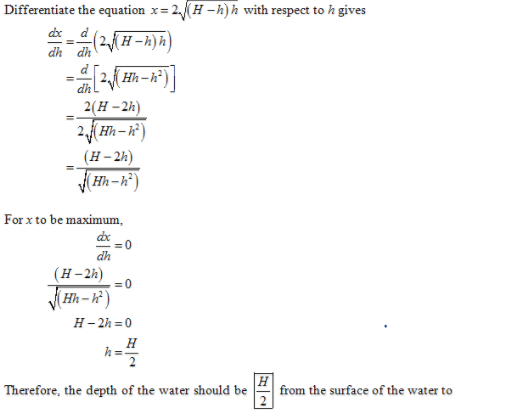JEE Main Practice Test- 26 - Question 82

A force 103 newton stretches the length of a hanging wire by 1 millimetre. The force required to stretch a wire of same material and length but having four times the diameter by 1 millimeter is

Detailed Solution for JEE Main Practice Test- 26 - Question 82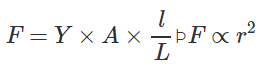(Y,l and L are constant) If diameter is made four times then force required will be 16 times. i.e. 16 x 103 N

JEE Main Practice Test- 26 - Question 83

The eye-piece used in biological microscope is

JEE Main Practice Test- 26 - Question 84

A ball rolls without slipping. The radius of gyration of the ball about an axis passing through its centre of mass is K. If radius of the ball be R, then the fraction of total energy associated with its rotational energy will be

Detailed Solution for JEE Main Practice Test- 26 - Question 84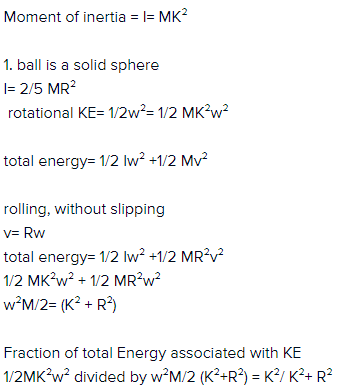JEE Main Practice Test- 26 - Question 85

We consider a thermodynamic system. If ∆U represents the increase in its internal energy and W the work done by the system, which of the following statements is true

Detailed Solution for JEE Main Practice Test- 26 - Question 85

∆Q=0
∆U=∆Q-∆W=0-W=-W.

JEE Main Practice Test- 26 - Question 86

A body of mass 5 g is executing S.H.M. with amplitude 10 cm. Its maximum velocity is 100 cm/s. Its velocity will be 50 cm/s at a displacement from the mean position equal to

Detailed Solution for JEE Main Practice Test- 26 - Question 86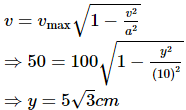JEE Main Practice Test- 26 - Question 87

The golden view of sea shell is due to

Detailed Solution for JEE Main Practice Test- 26 - Question 87

When a ray of light falls on sea shell, then its small amount first gets refracted (slightly polarised) and then almost gets reflected back (fully polarised). That is why, view of sea shell is golden due to polarisation.

JEE Main Practice Test- 26 - Question 88

The maximum speed of a body vibrating under simple harmonic motion with a time period of π/4 sec and amplitude of 7 cm is

JEE Main Practice Test- 26 - Question 89

Calcorimeters are made of which of the following

Detailed Solution for JEE Main Practice Test- 26 - Question 89

Calorimeters are made up of metal only because they are good conductor of heat and hence the heat exchange is quick which the basic requirement for the working of calorimeter.

JEE Main Practice Test- 26 - Question 90

The position of a particle at time 't' is given by the equation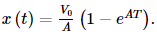Where V0 is constant and A > 0. Dimensions of V0 and A respectively are

Detailed Solution for JEE Main Practice Test- 26 - Question 90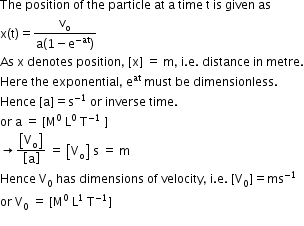## JEE Main & Advanced Mock Test Series

1 videos|356 docs|217 tests
Information about JEE Main Practice Test- 26 Page
In this test you can find the Exam questions for JEE Main Practice Test- 26 solved & explained in the simplest way possible. Besides giving Questions and answers for JEE Main Practice Test- 26, EduRev gives you an ample number of Online tests for practice

## JEE Main & Advanced Mock Test Series

1 videos|356 docs|217 tests